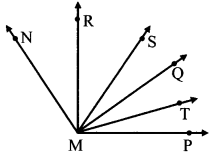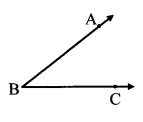# Maharashtra Board Practice Set 15 Class 7 Maths Solutions Chapter 4 Angles and Pairs of Angles

Balbharti Maharashtra State Board Class 7 Maths Solutions covers the 7th Std Maths Practice Set 15 Answers Solutions Chapter 4 Angles and Pairs of Angles.

## Angles and Pairs of Angles Class 7 Practice Set 15 Answers Solutions Chapter 4

Question 1.
Observe the figure and complete the table for ∠AWB.Points in the interior Points in the exterior Points on the arms of the angles

Solution:

 Points in the interior point C, point R, point N, point X Points in the exterior point T, point U, point Q, point V, point Y Points on the arms of the angles point A, point W, point G, point B

Question 2.
Name the pairs of adjacent angles in the figures below.Solution:
i. ∠ANB and ∠ANC
∠BNA and ∠BNC
∠ANC and ∠BNC

ii. ∠PQR and ∠PQT

Question 3.
Are the following pairs adjacent angles? If not, state the reason.1. ∠PMQ and ∠RMQ
2. ∠RMQ and ∠SMR
3. ∠RMS and ∠RMT
4. ∠SMT and ∠RMS

Solution:

1. ∠PMQ and ∠RMQ are adjacent angles.
2. ∠RMQ and ∠SMR not adjacent angles since they do not have separate interiors.
3. ∠RMS and ∠RMT not adjacent angles since they do not have separate interiors.
4. ∠SMT and ∠RMS are adjacent angles.

Maharashtra Board Class 7 Maths Chapter 4 Angles and Pairs of Angles Practice Set 15 Intext Questions and Activities

Question 1.
Observe the figure alongside and write the answers. (Textbook pg. no. 24)1. Write the name of the angle shown alongside___.
2. Write the name of its vertex___.
3. Write the names of its arms___.
4. Write the names of the points marked on its arms___.

Solution:

1. ∠ABC
2. Point B
3. Ray BA, ray BC
4. Points A, B, C